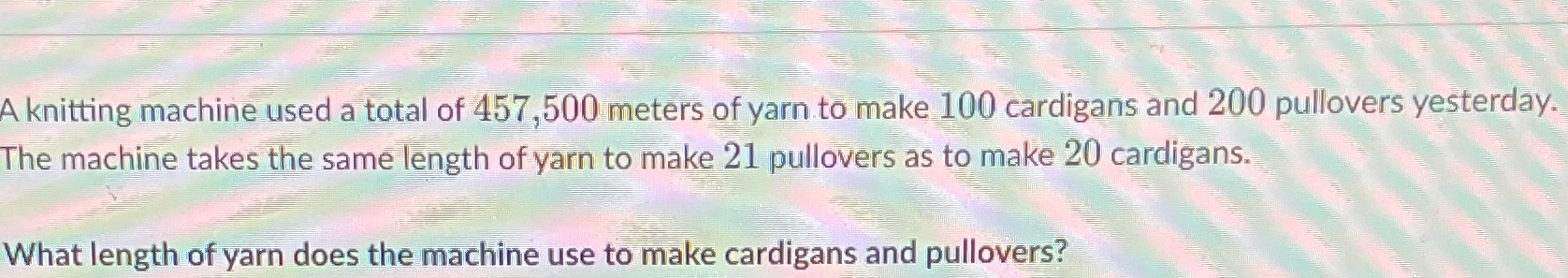### Still have math questions?

Algebra
QuestionA knitting machine used a total of $$457,500$$ meters of yarn to make $$100$$ cardigans and $$200$$ pullovers yesterday. The machine takes the same length of yarn to make $$21$$ pullovers as to make $$20$$ cardigans.

What length of yarn does the machine use to make cardigans and pullovers?